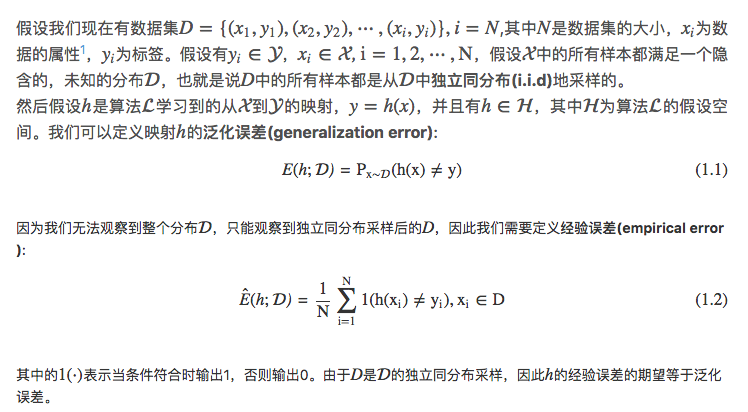• 泛化能力 一、 泛化误差 学习方法的泛化能力（generalization ability）：方法学习到的模型对未知数据...泛化误差定义：如果学习到的模型是f^\hat ff^​，那么用这个模型对未知数据预测的误差即为泛化误差（generali...
泛化能力
一、 泛化误差
学习方法的泛化能力（generalization ability）：方法学习到的模型对未知数据的预测能力。
评价标准：测试误差。
但因为测试数据集是有限的，很有可能由此得到的评价结果是不可靠的。统计学习理论试图从理论上对学习方法的泛化能力进行分析。

泛化误差定义：如果学习到的模型是$\hat f$，那么用这个模型对未知数据预测的误差即为泛化误差（generalization error）
$R_{exp}(\hat f)=E_p[L(Y,\hat f(X))]=\int_{\chi \times \gamma}L(y,\hat f(x))p(x,y)dxdy$
实际上，泛化误差就是学习到的模型的期望风险

意义：反映了学习方法的泛化能力，越小，则泛化能力越好

二、 泛化误差上界
泛化误差上界（generalization error bound）：泛化误差的概率上界。

通过比较两种学习方法的泛化误差上界的大小来比较它们的优势。

性质：

是样本容量的函数，当样本容量增加时，泛化上界趋于0
是假设空间容量的函数，假设空间容量越大，模型就越难学，泛化误差上界就越大。

二分类问题的泛化误差上界：
已知训练数据集$T=\{(x_1,y_1),(x_2,y_2),...,(x_N,y_N)\}$，它是联合概率分布$P(X,Y)$独立同分布产生的，$X\in R^n, Y\in \{-1,+1\}$. 假设空间是函数的有限集合$F=\{f_1,f_2,...,f_d\}$，d是函数个数。设f是从F中选取的函数。损失函数是0-1损失，关于f的期望风险和经验风险分别是：
$R(f)=E[L(Y,f(X))] \\ \hat R(f) = \frac{1}{N}\sum_{i=1}^N L(y_i,f(x_i))$
经验风险最小化函数是：
$f_N = arg \space min_{f\in F}\hat R(f)$
$f_N$的泛化能力：
$R(f_N)=E[L,f_N(X)]$
**定理(泛化误差上界)：**对二类分类问题，当假设空间是有限个函数的集合$F=\{f_1,f_2,...f_d\}$时，对任意一个函数$f\in F$，至少以概率$1-\delta$，以下不等式成立：
$R(f) \leq \hat R(f) + \epsilon(d,N,\delta) \tag{1}$
其中
$\epsilon(d,N,\delta)=\sqrt{\frac{1}{2N}(log\space d+log\frac{1}{\delta})}$
不等式（1）左端$R(f)$是泛化误差，右端即为泛化误差上界。在泛化误差上界中，第1项是训练误差，训练误差越小，泛化误差越小。第2项$\epsilon(d,N,\delta)$是N的单调递减函数，当$N$趋于无穷时，它趋于0；同时它也是$\sqrt{log\space d}$阶的函数，假设空间$F$包含的函数越多，其值越大。
**证明：**在证明中要用到$Hoeffding$不等式，先叙述如下
设$S_n=\sum_{i=1}^nX_i$是独立随机变量$X_1,X_2,...,X_n$之和，$X_i\in [a_i,b_i]$，则对任意$t>0$，以下不等式成立：
$P(S_n-ES_n\geq t) \leq exp(\frac{-2t^2}{\sum_{i=1}^n(b_i-a_i)^2}) \tag{2}$
$P(ES_n -S_n \geq t)\leq exp(\frac{-2t^2}{\sum_{i=1}^n(b_i-a_i)^2}) \tag{3}$
对任意函数$f\in F$，$\hat R(f)$是N个独立的随机变量$L(Y,f(X))$的样本均值，$R(f)$是随机变量$L(Y,f(X))$的期望值。如果损失函数取值于区间$[0,1]$，即对所有i，$[a_i,b_i]=[0,1]$，那么由$Hoeffding$不等式(3)不难得知，对$\epsilon>0$，以下不等式成立：
$P(R(f)-\hat R(f)\geq \epsilon) \leq exp(-2 N\epsilon^2)$
由于$F=\{f_1,f_2,...,f_d\}$是一个有限集合，故
$P(\exists f\in F:R(f)-\hat R(f)\geq \epsilon)=P(\bigcup_{f\in F}\{R(f)-\hat R(f) \geq \epsilon\}) \\ \leq \sum_{f\in F}P(R(f)-\hat R(f)\geq \epsilon) \\ \leq d \space exp(-2N\epsilon^2)$
或者等价的，对任意的$f\in F$，有
$P(R(f)-\hat R(f)< \epsilon) \geq 1-d \space exp(-2N\epsilon ^2)$
令
$\delta = d\space exp(-2N\epsilon^2) \tag{4}$
则
$P(R(f)<\hat R(f)+\epsilon) \geq 1-\delta$
则至少以概率$1-\delta$有$R(f)<\hat R(f)+\epsilon$<其中$\epsilon$由(4)得到，即为$\epsilon (d,N,\delta)$
从泛化误差上界可知，
$R(f_N)\leq \hat R(f_N)+\epsilon(d,N,\delta)$
其中$\epsilon(d,N,\delta)$和$f_N$在上面表示。因此，训练误差小的模型，其泛化误差也会小。
以上的讨论的只是假设空间包含有限个函数情况下的泛化误差上界，对一般的假设空间要找到的泛化误差界就没这么简单。


展开全文机器学习 统计学
• 泛化误差和经验误差 目录： - 基本概念 - 数学表达 - 应用 ------------------- 基本概念 西瓜书上的解释，学习器在训练集上的误差成为“训练误差”或“经验误差”。在新样本上的误差称为“泛化误差”。 以前...
泛化误差和经验误差

目录：
- 基本概念
- 数学表达
- 应用
-------------------

基本概念

西瓜书上的解释，学习器在训练集上的误差成为“训练误差”或“经验误差”。在新样本上的误差称为“泛化误差”。
以前在机器学习中泛化误差是用来衡量一个学习机器推广未知数据的能力，即根据从样本数据中学习到的规则能够应用到新数据的能力。

数学表达应用

根据大数定律，经验误差会收敛于泛化误差，两者（在一定容忍下）相近是由hoeffding不等式作为理论保证的，两者相差过大说明模型的欠拟合或者过拟合，而学习的一致收敛性说的正是这一特性：当训练集足够大，两者的结果就会足够相近，这样我们通过样本的“管中窥豹”的目的才能达到，最终才能获得一个目标假设。

参考资料

西瓜书，2.1，12.2，12.3


展开全文• 经验误差（empirical error）：也叫训练误差（training error），模型在训练集上的误差。 泛化误差（generalization error）：模型在新样本集（测试集）上的误差称为“泛化误差” ...
经验误差（empirical error）：也叫训练误差（training error），模型在训练集上的误差。

泛化误差（generalization error）：模型在新样本集（测试集）上的误差称为“泛化误差”

展开全文• 误差（error）：一般地，我们把学习器的实际预测输出与样本的真是输出之间的差异称为“误差” 经验误差（empirical ...泛化误差（generalization error）：模型在新样本集（测试集）上的误差称为“泛化误差”。 ...
误差（error）：一般地，我们把学习器的实际预测输出与样本的真是输出之间的差异称为“误差”

经验误差（empirical error）：也叫训练误差（training error）。模型在训练集上的误差。

泛化误差（generalization error）：模型在新样本集（测试集）上的误差称为“泛化误差”。

展开全文• 经验误差，泛化误差 前言我们在上篇博文 《机器学习模型的容量，过拟合与欠拟合》 中曾经提到过模型的泛化问题，指的就是描述一个模型在未见过的数据中的表现能力。这里再提出了，用于比较经验误差。 联系方式： ...机器学习 深度学习 人工智能
• 我们需要区分训练误差（training error）和泛化误差（generalization error）。通俗来讲，前者指模型在训练数据集上表现出的误差，后者指模型在任意一个测试数据样本上表现出的误差的期望，并常常通过测试数据集上的...机器学习 深度学习 K折交叉验证
• 今天我就来说说训练误差、测试误差、泛化误差到底是什么，区别所在。 对于分类学习算法，我们一般将样本集分为训练集和测试集，其中训练集用于算法模型的学习或训练，而测试集通常用于评估训练好的模型对于数据的...训练误差 测试误差
• 左端即为泛化误差，右端则为泛化误差上界。泛化误差也可以理解为期望风险，而右式第一个也叫做经验风险。 这都是与我们的的模型相关的，我们希望我们的模型对未知数据也能有好的预测能力，也就是泛化能力较强，这样...
• 在机器学习中，泛化误差（预测误差）是用于算法性能度量最常用的指标，然而由于数据的分布未知，泛化误差不能被直接计算，实际中常常通过各种形式的交叉验证方法来估计泛化误差。详细地分析了泛化误差的各交叉验证...机器学习 交叉验证
• 当train出来一个model之后，parameters已经定了下来。然后用test dataset去test这个network。 泛化误差就是test的时候的误差。
• 泛化误差 泛化即推广能力。 考虑在假设空间中的best function和可以从数据集中学到的best function之间的距离。 优化误差 因为优化问题带来的误差。 即从能数据集中学到的best function和使用该算法从数据集中学到的...误差分析 机器学习
• 个人对泛化误差的看法   泛化误差与交叉验证 误差这个词我们经常会遇到，在机器学习中，我们最终想要的结果实际上就是减小学习后的估计值和真实值的误差。比如在回归中，我们的 loss function 就表示一个误差。...交叉验证
• 后来业界就提出了泛化误差的概念（generalization error）,在机器学习中泛化误差是用来衡量一个学习机器推广未知数据的能力，即根据从样本数据中学习到的规则能够应用到新数据的能力。常用的计算方法是：用在训练集...
• 【统计学习笔记】泛化误差上界1. 泛化误差2. 泛化误差上界 1. 泛化误差 学习方法的泛化能力是指由该方法学习到的模型对未知数据的预测能力，是学习方法本质上重要的性质。测试误差是依赖于测试数据集的，泛化误差是...机器学习
• 基于支持向量机(SVM)泛化误差界,提出了一种精确且有效的多核学习方法.首先,应用SVM泛化误差界推导多核学习优化形式,并给出求解其目标函数微分的计算公式.然后,设计高效的迭代算法来求解该优化问题.最后,分析了算法的...
• 基于Rademacher复杂度的泛化误差界 待定。
• 泛化能力和泛化误差The center of any business is the customer. Understanding customer behavior and finding the most effective and appropriate way to accommodate their needs and expectations is the ...大数据
• 在建立一个分类模型的过程中我们暂时对检验数据集一无所知（也就是暂时得不到真正泛化误差），那么此时我们是如何估计泛化误差的？ 我们的目录： 目录 一、过拟合产生原因 二、过拟合与多重比较过程 三、泛化...过拟合
• 文章目录先导内容一、 泛化能力（generalization ability）二、 泛化误差（generalization error）三、泛化误差上界（generalization error bound）重点来了！霍夫丁不等式的证明一、Markov’s Inequality（马尔可夫...
• 一、期望泛化误差的偏差-方差分解 偏差-方差分解试图对机器学习算法的期望泛化误差率进行拆解。 记为测试样本，为训练集D上学习得到的模型在上的预测输出，为在数据集中的标记，为的真实标记。 对算法的期望泛化...机器学习
• 泛化误差：模型是f^\hat{f}f^​，该模型对于未知数据预测的误差即为泛化误差： Rexp⁡(f^)=EP[L(Y,f^(X))]=∫X×YL(y,f^(x))P(x,y)dx dy \begin{aligned} R_{\exp }(\hat{f}) &=E_{P}[L(Y, \hat{f}(X))] \\...机器学习
• 经验误差与泛化误差、偏差与方差、欠拟合与过拟合、交叉验证 主要内容 经验误差与泛化误差 偏差与方差 欠拟合与过拟合 交叉验证 一、经验误差（训练误差）与泛化误差   经验误差（训练误差）：模型在训练集上的...机器学习
• 参考人工智能前沿讲习的泛化误差专题 1、背景 训练集往往只是数据总体的一部分，无法包含所有可能的情况，训练出的学习算法在训练集和非训练集上的表现会是不一样的，我们使用泛化误差来度量这一差距，这也是机器...
• 介绍 本文详细推导了《统计学习方法》第一章中，泛化误差上界的不等式。其中利用到了《离散数学》、《概率论》等的知识 推导的详细过程 可能字迹不够工整，如果有问题可以发评论 ...机器学习 公式推导 人工智能
• 目录训练误差泛化误差总结 训练误差 训练误差（training error）：模型在训练数据集上表现出的误差 泛化误差 泛化误差（generalization error）：模型在任意一个测试数据样本上表现出的误差的期望，并常常通过测试...深度学习 机器学习 神经网络 人工智能
• 一般而言，我们将考虑训练集上的训练误差和测试集上的泛化误差，事实上，训练误差的持续降低并不是那么令人愉快，因为这可能是“过拟合”在背后操纵着一切。总的来说，只有泛化误差的降低才能真的让人感觉美滋滋。 ......